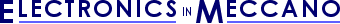www.eleinmec.com

The 555 Monostable Circuit
By Tim Surtell

In the previous article in this series we looked at the astable - a circuit in which the output has no stable state.  In this article we discuss the monostable circuit which has one stable state.

The waveforms in figure 1 illustrate the operation of a monostable.  A monostable circuit produces one pulse of a set length (time period T) in response to a trigger input such as a push button.  The output of the circuit stays in the low state until there is a trigger input, hence the name "monostable" meaning "one stable state".

This type of circuit is ideal for use in a "push to operate" system for a model displayed at exhibitions.  A visitor can push a button to start a model's mechanism moving, and the mechanism will automatically switch off after a set time.  Another use for the circuit is to 'de-bounce' a push button input to a digital IC - this application is explained later in this article.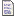Related Articles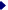The 555 Timer - Background information about the 555The 555 Astable Circuit - Includes an introduction to waveform terminologyThe 555 Bistable Circuit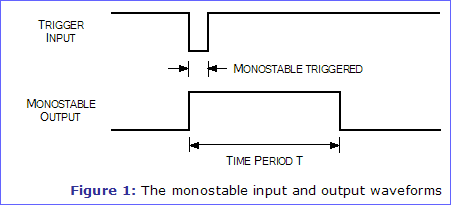#### The 555 Monostable Circuit

The circuit diagram of the 555 monostable circuit is given in figure 2.  Notice that the resistor value R and the capacitor value C are unspecified.  The values of these components determine the length of time that the monostable output is in the high state, and they may be calculated using the equation below...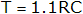...where T is the time period in seconds, and R and C are the component values in Ohms (Ω) and Farads (F).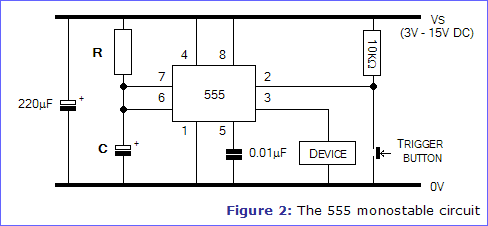##### Doing the Calculations

Here is a step-by-step guide to calculating the value of resistor R - an example is given in curly braces {}.

1. Firstly, decide the time period T that you require.  This can be very small (milliseconds) or large (minutes), but it must be expressed in seconds.  {I choose T = 10 seconds}
2. Next, guess a value for the capacitor C, expressed in Farads.  For starters, try 100μF.  {I choose C = 100μF}
3. Put the values of T and C into the equation below and calculator resistor R...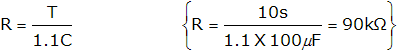If the resistor value you calculated is smaller than 1kΩ or larger than 1MΩ, you should re-do the calculation with a different value for capacitor C until you get a resistor value within the acceptable range.

##### Varying the Time Period

If you will need to adjust the time period of the monostable circuit in use, you can use a linear variable resistor for R, as shown in figure 3.

Because the resistance of a variable resistor goes down to around 0Ω at one end of its range, a 1kΩ resistor is placed in series with it so that the value of R will never fall below 1kΩ. As the shaft of the variable resistor is turned from its lowest setting to its highest, T will become longer.

If your chosen variable resistor has three connections, it is a potentiometer, and you should connect to the centre connection and either of the end connections.Further InformationResistors - Includes information about variable resistors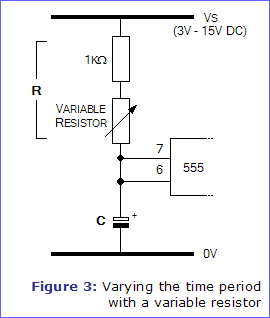##### The Trigger Input

As you can see from figure 1, the 555's Trigger input must be taken low to trigger the monostable.  This is achieved in figure 2 by placing a button in series with a resistor across the power supply.  Normally, the 10kΩ resistor keeps the Trigger input high, at the voltage Vs, and the monostable is in its steady state.  When the button is pushed, the Trigger input is directly connected to 0V and the time period T starts.

##### The Reset Input

If you want to make the monostable output go low before the time period has elapsed, simply take the 555's Reset input briefly low.  This can be achieved with a push button in exactly the same way as with the Trigger input.

#### De-bouncing

When a push button is pressed or released, the contacts will bounce between the on and off states for a fraction of a second.  If the button is connected to the input of a digital IC, then the IC will think the button is being pressed several times rather than once.  While this may not matter in some circuits like the monostable, to others it will produce erratic behaviour.

For example, if the button is connected to the Clock input of a digital counter IC, the counter output should increment once every time the button is pressed.  Instead it will count perhaps 5 times due to the contact bounce in the button.

To solve the problem, the push button must be 'de-bounced' by placing a small delay between it and the digital input - an ideal application for a monostable.  Figure 4 shows a block diagram of this arrangement.  Obviously, there is a need to get the length of the delay (the time period) correct - too short and some of the bounce will get through, too long and you will prevent quick successions of button presses having an effect on the digital input.  A suitable time period for most applications would be around 100ms.  To make a 555 monostable with this time period you would need to make R = 91kΩ and C = 1μF.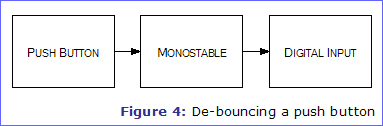#### Building the Circuit

A stripboard layout for the 555 monostable circuit is given in figure 5.  It provides a direct output from the 555 (pin 3), but if you want the monostable to control another device, such as a motor, you will probably want to leave some extra board space for any extra components required.  Some suggestions are given in "Using the 555 output" from part 1 of this series, The 555 Timer.

The 220μF capacitor is included to smooth the power supply, and can be omitted if you are using a regulated supply or the circuit functions correctly without it.

##### Construction
1. Cut a piece of stripboard to 12 tracks x 21 columns + extra columns for any extra components required.
2. Fit the 5 wire links.
3. Fit the 10KΩ resistor and the resistor R.
4. Fit the 8-pin IC socket, taking care to align the notch as shown in the layout.  Do not insert the 555 at this time.
5. Fit the 0.01μF, 220μF capacitors and timing capacitor C.  Ensure that the polarity of the 220μF capacitor is correct.  The leads will be marked with '+' or '-'.  The timing capacitor you have chosen could also be polarised, and should also be carefully placed.
6. Fit the components associated with the device that the 555 is controlling.
7. Insert the 555 into its socket, taking care to align the notch with the notch in the socket.
8. Cut the copper tracks where an X is shown.
9. Connect up a 3V - 15V DC power supply and test the circuit.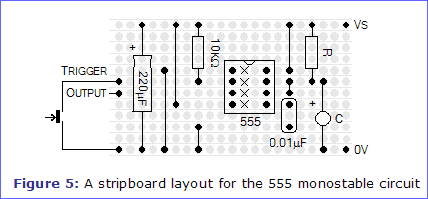Article Information
Source: Electronics in Meccano - www.eleinmec.com | First published in EiM: Issue 4 (June 1999)
Topic: Digital Electronics | Created: 5/14/2002 | Last modified: 2/15/2007Hosted on a Memset Dedicated Server | © 1998 - 2022 Tim Surtell